# 3 - Slope Design

This tutorial describes how to design a slope to Eurocode 7 specifications using RocTopple. The focus is on how different design combinations are applied in the computation and what they mean.

Topics Covered in this Tutorial:

• Eurocode 7 Design Standards
• Partial Factors
• Building Equivalent Models

Finished Product:

The finished model can be found in the Tutorial 3 Eurocode Design.rtop data file, located in the Examples > Tutorials folder in your RocTopple installation folder.

## 1.0 Introduction

Eurocode 7 is a design document that establishes rules and standards for geotechnical engineering design across Europe (BSI, 2004). Eurocode 7 represents a major change in design philosophy. Traditionally a single, lumped Factor of Safety accounts for all of the uncertainties in the problem. With Eurocode 7, partial Factors of Safety are applied to different components of the analysis. The partial factors are applied prior to the analysis to give design values that are used in the calculation. The final result is an over-design factor, which must be greater than 1 to ensure the serviceability limit state requirement is satisfied. For more information on using Eurocode 7 in geotechnical design, see Smith (2006) and Bond and Harris (2008).

## 2.0 Creating a New File

1. Start RocTopple by selecting Programs > Rocscience > RocTopple > RocTopple from the Windows Start menu.

RocTopple automatically opens a new blank document, which allows you to begin creating a model immediately. If the RocTopple application window is not already maximized, maximize it now so the full screen space is available for use.

If you do NOT see a model on your screen:

1. Select: File > New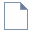Whenever a new file is created, the default input data will form valid slope geometry, as shown in the figure below: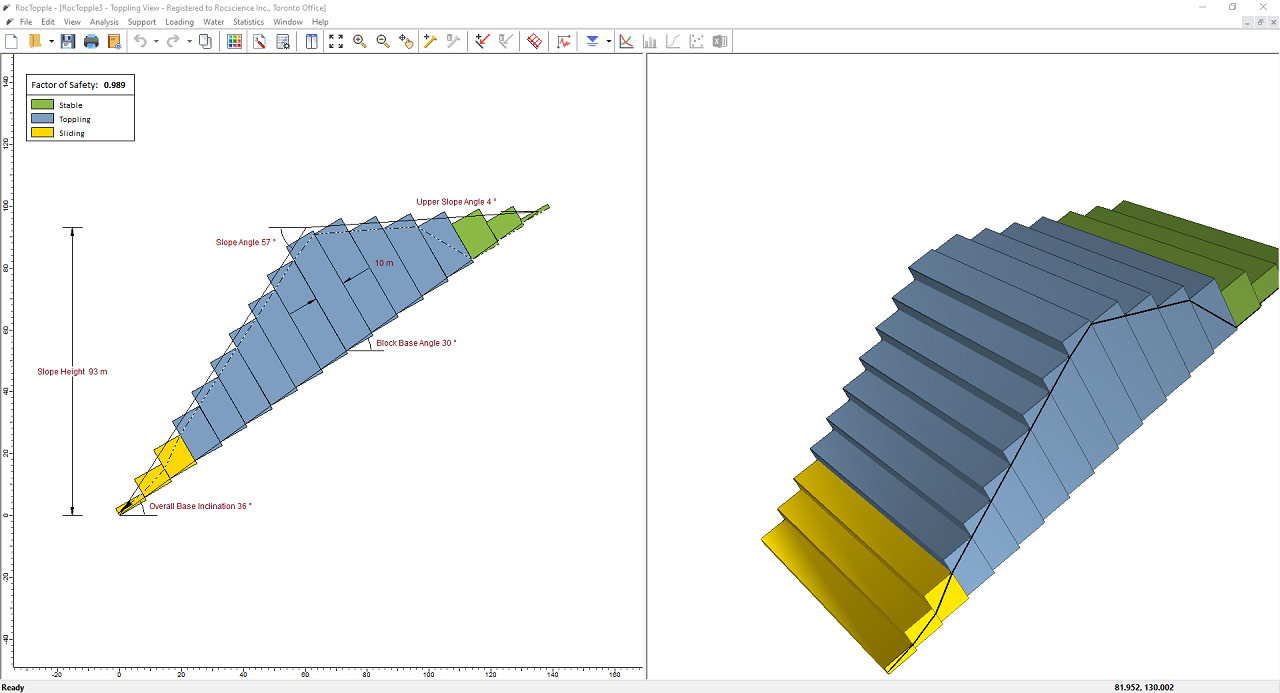Notice the split screen format of the display. The 2D View shows the slope geometry and the factor of safety, while the 3D View is interactive. Note that the computation is for two-dimensional geometry and assumes unit depth.

## 3.0 Model

### 3.1 PROJECT SETTINGS

Let's start by defining the settings for the project in the Project Settings dialog. To open the dialog:

1. Select: Analysis > Project Settings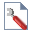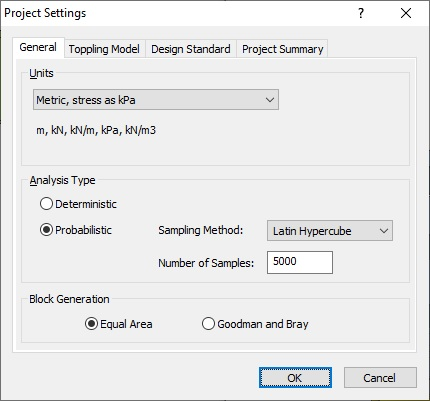#### 3.1.1 Units

For this tutorial, we will use Metric, stress as kPa as the Units.

#### 3.1.2 Design Standard

1. Select the Design Standard tab.
2. Keep Design Standard = None. We'll come back to this option later in the tutorial.
3. Click View Partial Factors.
Note that, with no Design Standard set, all partial factors are set to the default of 1, which means all values used in the calculation are the exact input values.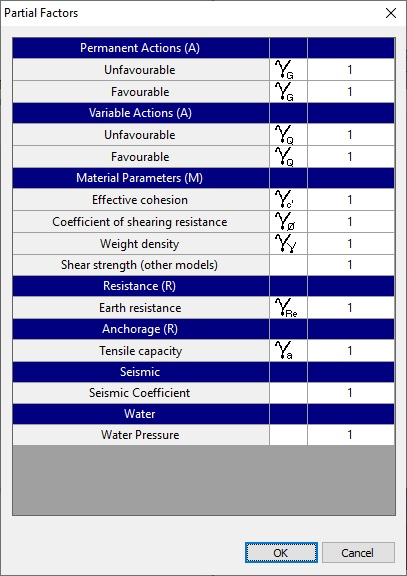4. Click Cancel to close the Partial Factors dialog.
5. Click OK to close the Project Settings dialog.

### 3.2 INPUT DATA

Next we'll enter input data using the Input Data dialog. To open the dialog:

1. Select: Analysis > Input Data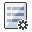2. In the Geometry tab, enter the values as shown in the figure below.
3. Slope

• Angle (degrees) = 83
• Height (m) = 93

Upper Slope

• Angle (degrees) = 0

Toppling Joints

• Spacing (m) = 8
• Dip (degrees) = 80

Overall Base Inclination

• Angle (degrees) = 50
• Rock Unit Weight (kN.m3) = 27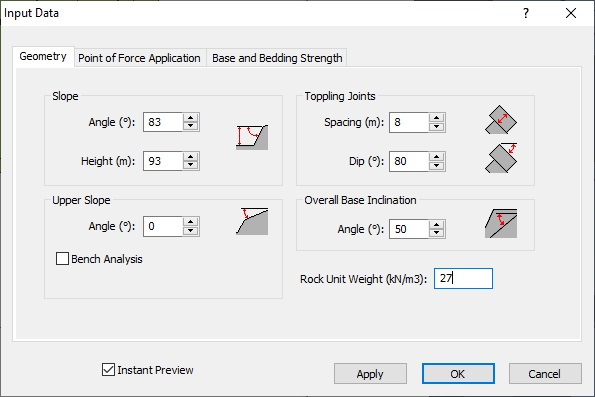4. Select the Base and Bedding Strength tab and enter the values as shown in the figure below.
5. Base Joints

• Shear Strength Model = Mohr-Coulomb
• Friction Angle (degrees) = 40
• Cohesion (kPa) = 0
• Tensile Strength (kPa) = 0

Toppling Joints

• Shear Strength Model = Mohr-Coulomb
• Friction Angle (degree) = 33
• Cohesion (kPa) = 0
• Tensile Strength (kPa) = 0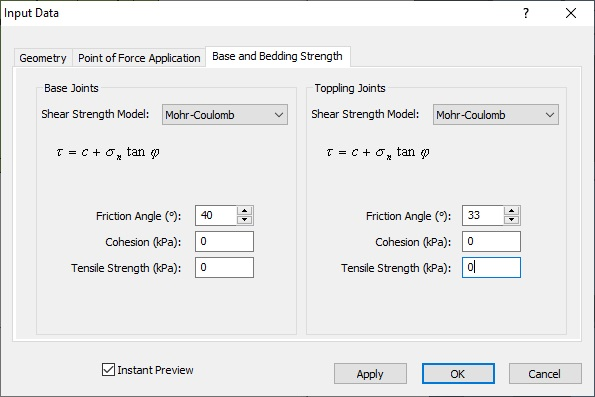6. Click OK to save changes and close the dialog.

The model should now have a Factor of Safety of 0.444, with blocks 1 – 6 toppling, and blocks 7 – 12 stable (at point of failure).

### 3.3 SUPPORTS

Now let’s add a bolt to the toe block, using the Add Spot Bolt dialog. To open the dialog:

1. Select: Support > Add Bolt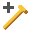2. In the dialog, enter the bolt specifications as shown in the figure below.
• Name = Bolt 1

Strength

• Capacity (kN) = 500
• Out-of-Plane Spacing (m) = 0.5

Geometry

• Length (m) = 32
• Angle (degree) = 0

Installation

• Slope Face
• Height above Toe (m) = 12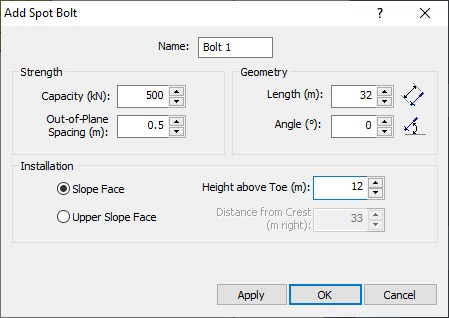3. Click OK.
4. Add another bolt as above, but set Geometry Length (m) = 40 and Height above Toe (m) = 39.
5. Click OK.

The Factor of Safety should now be 0.708, as shown in the figure below.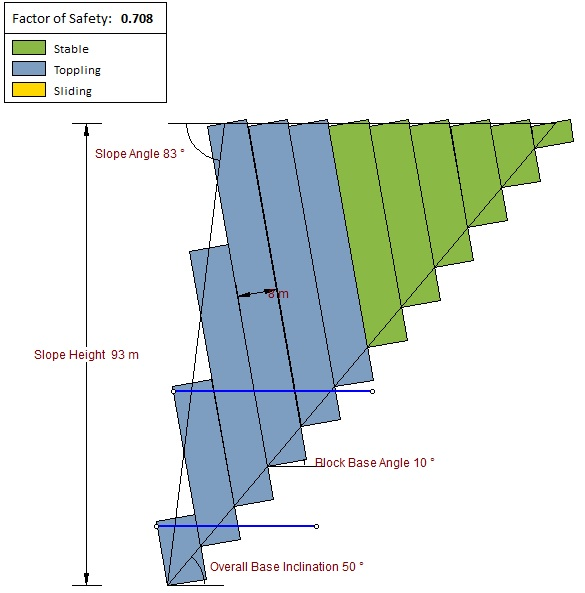## 4.0 Design Approach 1, Combination 2

### 4.1 APPLY THE STANDARD

Without changing the current input data, let’s go back to the Project Settings dialog to apply a design standard.

1. Re-open the Project Settingsdialog.
2. Select the Design Standard tab.
3. In the Design Standard drop-down, select Eurocode 7 – Design Approach 1, Combination 2.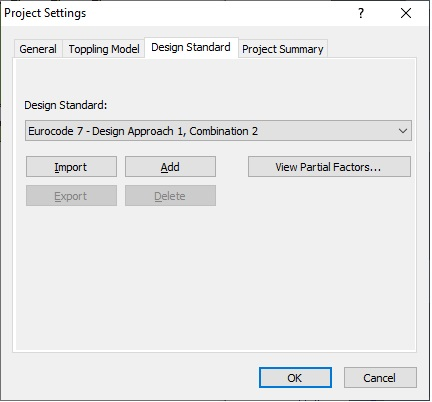4. Click View Partial Factors to view the partial factors for the selected standard.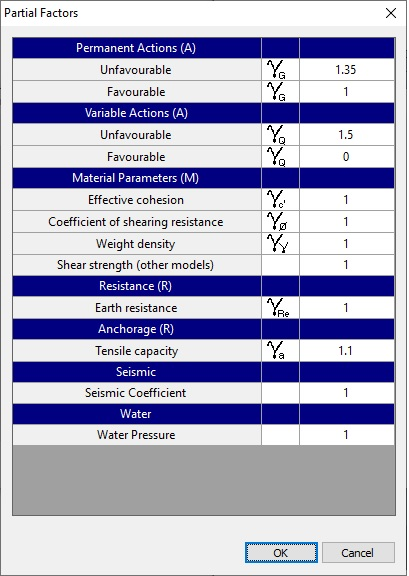Applying this set of partial factors means enlarging unfavorable variable actions while ignoring favorable variable actions, and reducing the shear strength parameters. If bolts are present, their capacity or the resistance they provide to stabilize the slope is also reduced.
5. Click OK in the Partial Factors dialog to close it.
6. Click OK in the Project Settings dialog.

The model should now have a Factor of Safety of 0.53. At the point of failure, blocks 1 – 6 are toppling, and 7 – 12 are stable.

### 4.2 EQUIVALENT MODEL

We can reproduce the results from Design Standard Approach 1, Combination 2, without having to use the Design Standard option.

Before continuing, re-set Design Standard to None in the Project Settings dialog as shown above. This should revert the Factor of Safety back to 0.708.

To simulate the design approach, we need to apply a factor to the following parameters:

• Increase variable unfavorable actions by a factor of 1.3. We do not need to change anything because there are no external variable loads.
• Reduce the effective cohesion and the friction angle (tan(φ)) by a factor of 1.25.

First, make the following changes to the input data:

1. Re-open the Input Datadialog.
2. Select the Base and Bedding Strength tab.
3. Set Base Joints Friction Angle = 33.87 and Toppling Joints Friction Angle = 27.45.
Base Joints Friction Angle: tan-1(tan(40°)/1.25) = 33.87°
Toppling Joints Friction Angle: tan-1(tan(33°)/1.25) = 27.45°
4. Click OK to close the dialog.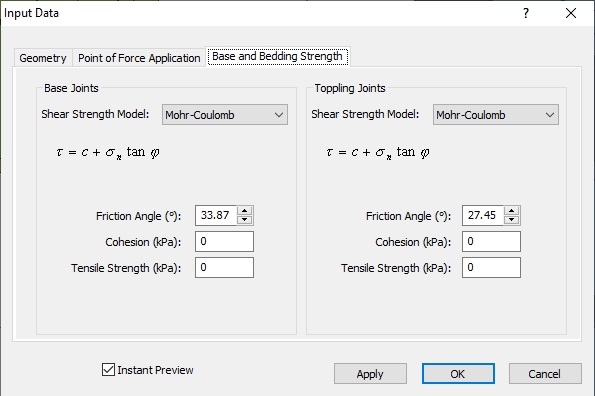Next, reduce the tensile capacity of the bolts we added earlier:

1. Right-click the bolt in the 2D View and select Edit Bolt in the popup.
The Edit Spot Bolt dialog appears.
2. Reduce the Strength Capacity to 454kN (500kN/1.1 = 454kN).
3. Click OK.
4. Repeat the steps above to edit the second bolt.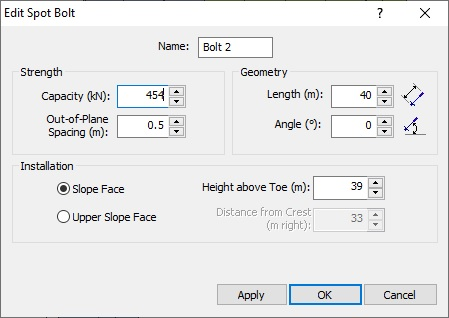The Factor of Safety should now be 0.53, which is exactly the same as that for Design Approach 1, Combination 2. You should also see that at the point of failure, blocks 1 – 6 are toppling, while 7 – 12 are stable.

## 5.0 Design Approach 2

Let's now apply a second design standard. We'll keep the existing geometry but delete the two bolts. We'll also add a line load.

### 5.1 CHANGE THE MODEL

To delete the bolts:

1. Select Delete Bolt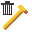on the toolbar or the Support menu.
The cursor should turn into a square.
2. Click both bolts to select them (highlighted).
3. Right-click and select Done.

Change the model's input data:

1. Re-open the Input Datadialog.
2. Select the Base and Bedding Strength tab
3. Set Base Joints Friction Angle = 40 and Toppling Joints Friction Angle = 33.

The Factor of Safety should be 0.444.

1. Select Add Line Loadon the toolbar or the Loading menu.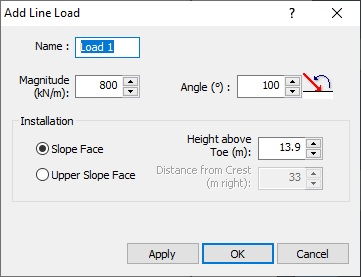3. Click OK.

The Factor of Safety should have increased to 0.472. This is because the line load on the toe block provides a resisting moment to the block.

### 5.2 APPLY THE STANDARD

Now we'll apply the standard.

1. Re-open the Project Settingsdialog.
2. Select the Design Standard tab.
3. In the Design Standard drop-down, select Design Standard to “Eurocode - Design Approach 2.
4. Click View Partial Factors.
You should see the following dialog: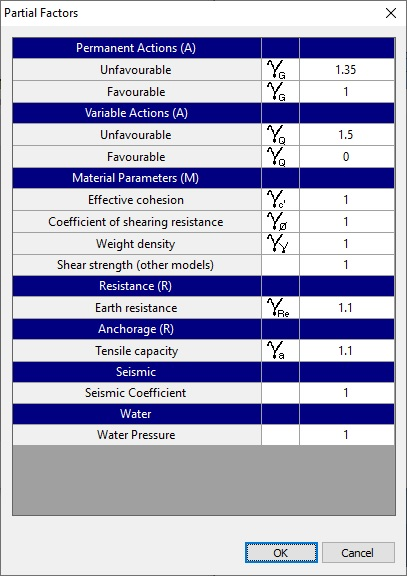For this design standard, a partial factor would be applied to the driving force of the slope weight (permanent unfavorable action), any unfavorable external force that is set to be “variable,” the joint shear strength, and the tensile capacity of any bolts.
5. Click OK in the Partial Factors dialog.
6. Click OK in the Project Settings dialog.

The Factor of Safety should now have decreased to 0.404. Blocks 1 – 6 should be toppling at the point of failure, while blocks 7 – 12 should be stable.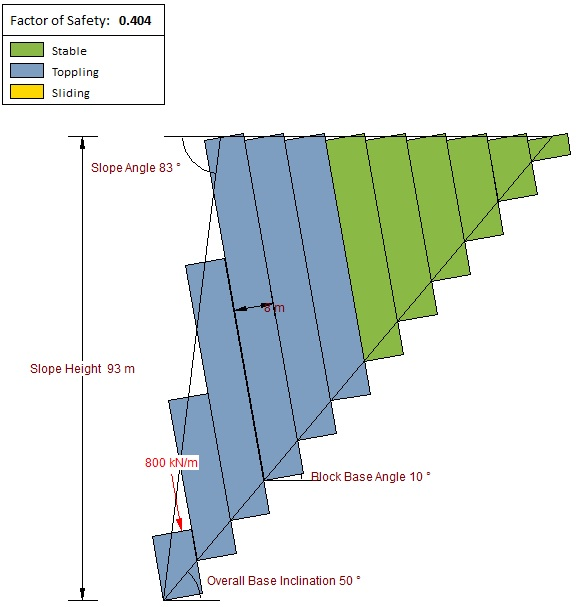### 5.3 EQUIVALENT MODEL

We can construct a model that has the same effects and would produce the same results as when partial factors are applied with Eurocode 7 - Design Approach 2.

Before continuing, make sure Design Standard is set to None. The Factor of Safety should revert to 0.472.

To simulate the design approach, apply changes to the following parameters:

1. Under the Geometry tab in the Input Data dialog, multiply the Rock Unit Weight by 1.35, to get 36.45kN/m3.
This has the same effect as applying a factor of 1.35 to any permanent unfavorable actions, which in this case is the driving force due to the slope weight. However, remember that the weight has both a favorable and unfavorable action component (stabilizing and driving). We have to adhere to the Single Source Principle and apply only 1 factor to any actions that comes from the same source. The Factor of Safety should now be 0.464.
2. Under the Base and Bedding Strength tab in the Input Data dialog, we need to reduce the overall joint shear strength by a factor of 1.1. This is the equivalent to reducing the Earth Resistance by a factor of 1.1.
a. Base Joints Friction Angle: tan-1(tan(40°)/1.1) = 37.34°
b. Toppling Joints Friction Angle: tan-1(tan(33°)/1.1) = 30.56°
Keep in mind that this is different from reducing individual shear strength parameters. If cohesion is present, then a factor of 1.1 is applied to the overall shear strength:
Factored Shear Strength = (Normal Force x Tan(φ) + cohesion)/1.1
Click OK in the Input Data dialog. The Factor of Safety should now be 0.422.
3. We need to Delete the Line Load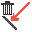. This is because a partial factor of 0 is applied to any favorable variable action in Approach 2. The current Line Load is a favorable action because it provides a resisting moment on the toe block. Also, Line Loads by default are set to be “variable” actions whenever a design standard is applied (you have the option to change a Line Load to be a “permanent” action in the Add/Edit Line Load dialog).

To delete the Line Load, right-click on the Line Load and select Delete.

The Factor of Safety is now 0.404, the same as for Eurocode – Design Approach 2.

NOTE: For more help on Eurocode design, see Design Standard Setting in RocTopple.

This concludes this tutorial. You are now ready for the next tutorial, Tutorial 04 - RocTopple Export to RS2.